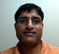# Computing an FFT of Complex-Valued Data Using a Real-Only FFT Algorithm

Someone recently asked me if I knew of a way to compute a fast Fourier transform (FFT) of complex-valued input samples using an FFT algorithm that accepts only real-valued input data. Knowing of no way to do this, I rifled through my library of hardcopy FFT articles looking for help. I found nothing useful that could be applied to this problem.

After some thinking, I believe I have a solution to this problem. Here is my idea:

Let's say our original input data is the complex-valued sequence xc(n) having N samples, and the desired FFT of that complex xc(n) sequence is the complex-valued sequence Xc(m), where the time and frequency indices are 0≤nN–1 and 0≤mN–1 respectively. The input xc(n) can be represented by:

xc(0) = xr(0) + jxi(0),
xc(1) = xr(1) + jxi(1),
xc(2) = xr(2) + jxi(2),
...
...
xc(N-1) = xr(N-1) + jxi(N-1).    (1)

If the result of a real FFT of the real part of xc(n), xr(n), is Xr(m), and the result of a real FFT of the imaginary part of xc(n), xi(n), is Xi(m), then the desired complex FFT of xc(n) is:

Xc(m) = real[Xr(m)] - imag[Xi(m)] + j{imag[Xr(m)] + real[Xi(m)]}    (2)

where j = sqrt(–1).

I don't claim that Eq. (2) is novel, or special, in any way. (There are probably 8,000 guys out there who've already solved this problem.) I merely present Eq. (2) here because I haven't seen it anywhere else. Who knows, maybe Eq. (2) will be of use to someone out there. An algebraic justification for Eq. (2) is given in the Appendix.

APPENDIX
There exists a technique where two independent N–point real input data sequences can be transformed using a single N–point complex FFT. We call this scheme the "Two N–Point Real FFTs" algorithm. The derivation of this technique is straightforward and described in the literature. – If two N–point real input sequences are xr(n) and xi(n), they'll have FFTs represented by Xr(m) and Xi(m). If we treat the xr(n) sequence as the real part of a complex FFT input and the xi(n) sequence as the imaginary part of the complex FFT input, then the constructed complex xc(n) input sequence to a complex FFT is:

xc(0) = xr(0) + jxi(0),
xc(1) = xr(1) + jxi(1),
xc(2) = xr(2) + jxi(2),
...
...
xc(N-1) = xr(N-1) + jxi(N-1).    (A-1)

If the N-point FFT of xc(n) is Xc(m), then the desired Xr(m) and Xi(m) results are:

Xr(m) = [Xc(N–m)* + Xc(m)]/2    (A-2)

and

Xi(m) = j[Xc(N–m)* – Xc(m)]/2    (A-3)

where 0≤m≤N-1, and the "*" symbol means complex conjugate. Equations (A-2) and (A-3) are well known.(Due to the periodicity of Xc(m), when m = 0, Xc(N-m)* = Xc(0)*.)

But our problem is the reverse of the above expressions: in this blog we know Xr(m) and Xi(m) and we desire to find Xc(m). We do that by rearranging Eqs. (A-2) and (A-3), solving them for Xc(N-m)*, and writing:

2Xr(m) - Xc(m) = Xc(Nm)*    (A-4)

and

2Xi(m)/j + Xc(m) = Xc(Nm)*.    (A-5)

Setting the left sides of Eqs. (A-4) and (A-5) equal to each other and solving for Xc(m), we have:

Xc(m) = Xr(m) - Xi(m)/j .    (A-6)

We're almost finished. Converting Eq. (A-6) into the more convenient rectangular form, we write

Xc(m) = real[Xr(m)] +j•imag[Xr(m)] -{real[Xi(m)] +j•imag[Xi(m)]}/j    (A-7)

or

Xc(m) = real[Xr(m)] +j•imag[Xr(m)] -{imag[Xi(m)] - j•imag[Xi(m)]}    (A-8)

Combining the real and imaginary terms in Eq. (A-8), we (finally) have our desired:

Xc(m) = real[Xr(m)] - imag[Xi(m)] + j{imag[Xr(m)] + real[Xi(m)]}    (A-9)

which is equivalent to this blog's Eq. (2).Cooley, J., Lewis, P., and Welch, P. “The Fast Fourier Transform Algorithm: Programming Considerations in the Calculation of Sine, Cosine and Laplace Transforms,” Journal Sound Vib., Vol. 12, July 1970, pp. 315-337.
 Brigham, E. The Fast Fourier Transform and Its Applications, Prentice Hall, Englewood Cliffs, New Jersey, 1988, pp. 166-167.
 Burrus, C. et al., Computer-Based Exercises for Signal Processing, Prentice Hall, Englewood Cliffs, New Jersey, 1994, pp. 56.
 Lyons, R. Understanding Digital Signal Processing, 2nd Ed., Prentice Hall, Englewood Cliffs, New Jersey, 2004, pp. 488-490.

[ - ]
Comment by February 11, 2010Am I missing something or did you just use a very complicated way to describe the linearity of the Fourier transform? xc = xr + j*xi <=> Xc = Xr + j*Xi <=> Xc = real(Xr) + j*imag(Xr) + j*(real(Xi) + j*imag(Xi)) <=> Xc = real(Xr) - imag(Xi) + j*(real(Xi) + image(Xr))
[ - ]
Comment by February 11, 2010Hello unconed, As it turns out, yes, you are correct. Although I didn't arrive at my Eq. (2), in the way I did, in order to make the problem appear complicated. I merely did not, for some reason, see the simple linearity property that you did unconed. You've given me another example of: how we view a problem, how we understand a problem, profoundly affects how we try to solve a problem. I knew my Eqs. (A-2) and (A-3) and thought, "How do I find xc(m)?" This isn't the first time I've solved a problem "the hard way." I wonder if it'll be the last time. Thanks unconed. [-Rick-]
[ - ]
Comment by February 16, 2010Along similar lines, there is also a neat trick to compute the FFT of a real-valued signal given an FFT that accepts only complex-valued input. This situation is actually encountered quite frequently on DSP platforms.

To post reply to a comment, click on the 'reply' button attached to each comment. To post a new comment (not a reply to a comment) check out the 'Write a Comment' tab at the top of the comments.